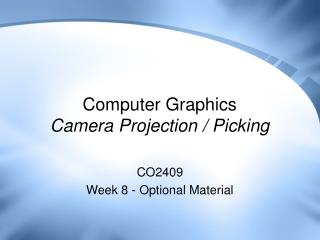DownloadDownload PresentationComputer Graphics Camera Projection / Picking

# Computer Graphics Camera Projection / Picking

Download Presentation## Computer Graphics Camera Projection / Picking

- - - - - - - - - - - - - - - - - - - - - - - - - - - E N D - - - - - - - - - - - - - - - - - - - - - - - - - - -
##### Presentation Transcript

1. Computer GraphicsCamera Projection / Picking CO2409 Week 8 - Optional Material

2. Contents • World / View Matrices Recap • Projection Maths • Pixel from World-Space Vertex • World Space Ray from Pixel • These notes are presented as additional material and are not examinable

3. Model Space • An model’s mesh is defined in its own local coordinate system - model space • Each model is positioned with a matrix • Transforming it from model space into world space • This matrix is called the World Matrix

4. World to Camera Space • Next consider how the models are positioned and oriented relative to the camera • Convert the models from world space into camera space • The scene as viewed from camera’s position • This transformation is done with the view matrix

5. Camera to Viewport Space • Finally project the camera space models into 2D • The 3D vertices are projected to camera position • Assume the viewport is an actual rectangle in the scene • Calculate where the rays hit the viewport = 2D geometry • This is done with the projection matrix

6. Projection Details • Cameras have two settings: • Field of View (FOV) • Viewport distance (D) • Viewport distance is same as the near clipping plane • Where geometry “slices” through the viewport • FOV works as a wide angle or zoom lens • FOV can be different for width and height – FOVX & FOVY

7. Projecting a Vertex • Consider the projection of a single 3D vertex to 2D • Want 2D coordinates (XV, YV) • YV not shown in diagram • Calculate using similar triangles: X / Z = XV / D, so XV = D * X / Z In a similar way, YV = D * Y / Z This is the perspective divide • Now have 2D coords, but still in camera space units • Need to convert to pixels

8. Converting to Pixels • Calculate the actual viewport dimensions (camera space): tan(FOVX / 2) = (WV / 2) / D so WV = 2 * D * tan(FOVX / 2) similarly, HV = 2 * D * tan(FOVY / 2) • Then calculate: XN = 2 * XV / WV YN = 2 * YV / HV • This 2D coordinate(XN, YN) is in the range –1 to 1 • Ready to convert to pixel position

9. Converting to Pixels • If the viewport width & height (in pixels) are WP and HP: then XP= (XN + 1) WP / 2 and YP= (1 - YN) HP / 2 • The second formula flips the Y axis (viewport Y is down) • (XP, YP) are the coordinates of the final pixel we want

10. Picking • Sometimes we need to manually perform the projection process: • To find the pixel for a particular 3D point • E.g. To draw text/sprites in same place as a 3D model • Or perform the process in reverse: • Each 2D pixel corresponds to a ray in 3D space (refer to the projection diagram) • Finding and working with this ray is called picking • E.g. to find the 3D object under the mouse • The algorithms for both follow – they are derived from the previous slides

11. Pixel from World-Space Vertex • Start with world space vertex P • Transform this vertex by combined view / projection matrix to give Q • If Q.z < 0 then the vertex is behind us, discard • Otherwise do perspective divide: Q.x /= Q.z and Q.y /= Q.z • Finally, scale to pixel coordinates X,Y: X = (Q.x + 1) * (ViewportWidth / 2) Y = (1 - Q.y) * (ViewportHeight / 2) • Use to draw text/sprites in same place as 3D entity

12. World-Space Ray From Pixel 1 • Initial pixel (X,Y), first convert to point Q in the range -1 -> 1: Q.x = (2 * X / ViewportWidth) - 1 Q.y = 1 – (2 * Y / ViewportHeight) • Set Q.z = D (viewport / near clip distance) • The result vertex will be exactly on the clip plane • Calculate viewport size in camera space: WV = 2 * D * tan(FOVX / 2) HV = 2 * D * tan(FOVY / 2) • If FOVY not available: HV = WV * ViewportHeight / ViewportWidth

13. World-Space Ray From Pixel 2 • Convert Q into camera space: Q.x *= WV / 2 Q.y *= HV / 2 • Finally transform by the inverse of the view matrix to get a point in world space • Then cast a ray from camera to this point • Use this 3D ray to detect the entity at the pixel